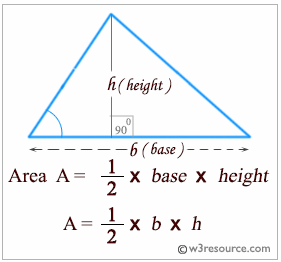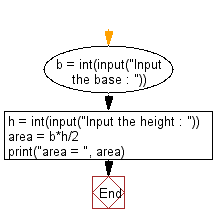﻿ Python: Accept the base and height of a triangle and compute the area - w3resource# Python: Accept the base and height of a triangle and compute the area

## Python Basic: Exercise-30 with Solution

Write a Python program that will accept the base and height of a triangle and compute the area.

Python: Area of a triangle

A triangle is a polygon with three edges and three vertices. It is one of the basic shapes in geometry. A triangle with vertices A, B, and C is denoted triangle ABC.

• Vertex of a triangle: The point at which two sides of a triangle meet.
• Altitude of a triangle: The perpendicular segment from a vertex of a triangle to the line containing the opposite side.
• Base of a triangle: The side of a triangle to which an altitude is drawn.
• Height of a triangle: The length of an altitude.Sample Solution:-

Python Code:

``````b = int(input("Input the base : "))
h = int(input("Input the height : "))

area = b*h/2

print("area = ", area)
```
```

Sample Output:

```Input the base : 20
Input the height : 40
area =  400.0
```

Flowchart:## Visualize Python code execution:

The following tool visualize what the computer is doing step-by-step as it executes the said program:

Python Code Editor:

Have another way to solve this solution? Contribute your code (and comments) through Disqus.

What is the difficulty level of this exercise?

Test your Python skills with w3resource's quiz

﻿

## Python: Tips of the Day

List comprehension:

```>>> m = [x ** 2 for x in range(5)]
>>> m
[0, 1, 4, 9, 16]
```